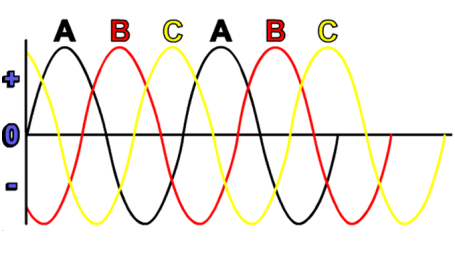Interactive

# Fundamentals of Three-Phase AC

Martech
Updated Jan 21, 2021

At the end of this lesson, you will be able to identify the construction of an AC circuit, calculate the circuits’ capacity value, and conduct power factor corrections in AC circuits. • Describe the differences between three-phase and single-phase voltages • Describe the characteristics of delta and wye connections • Calculate current and voltage for delta-connected circuits • Calculate current and voltage for wye-connected circuits • Describe the purpose of power factor • Calculate capacitance needed to make a power factor correction

;